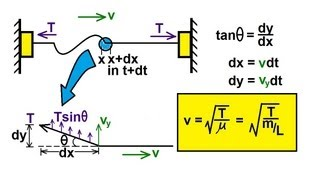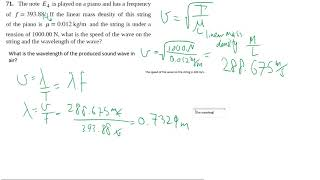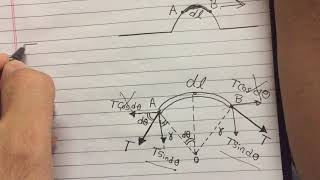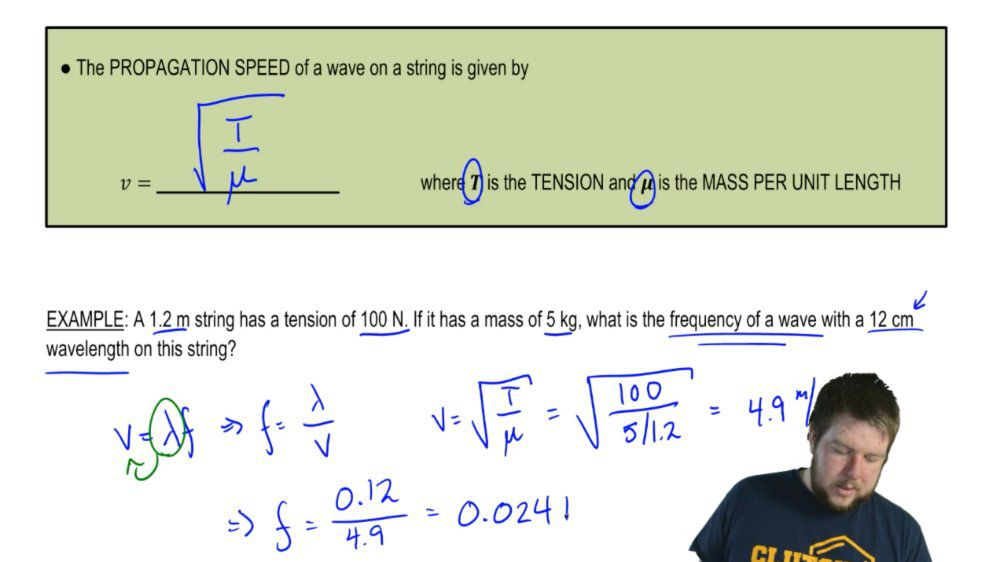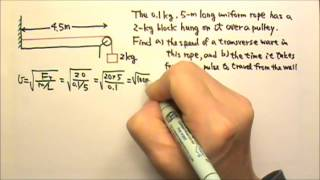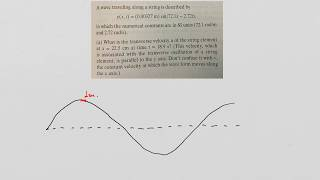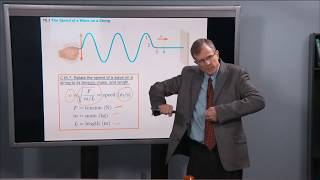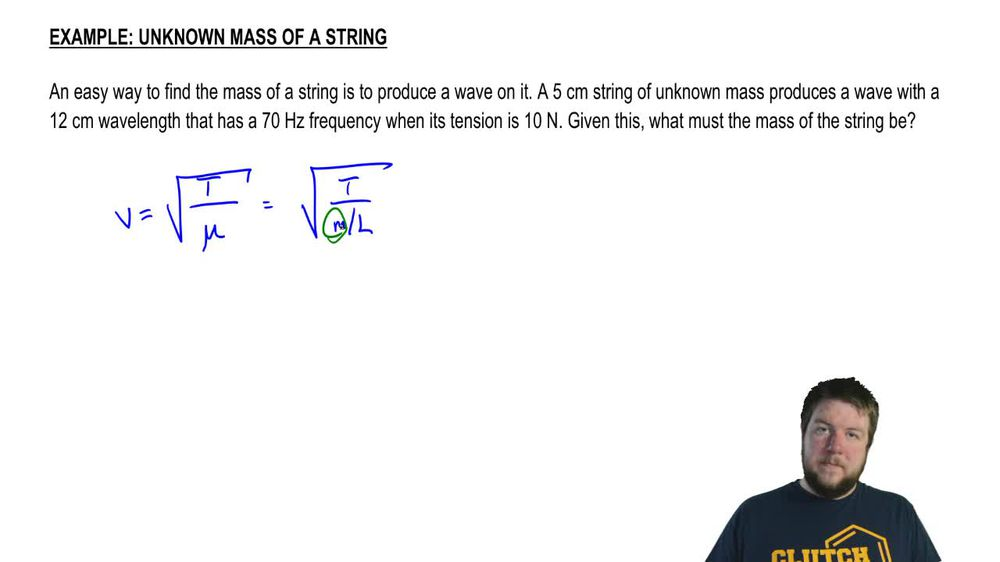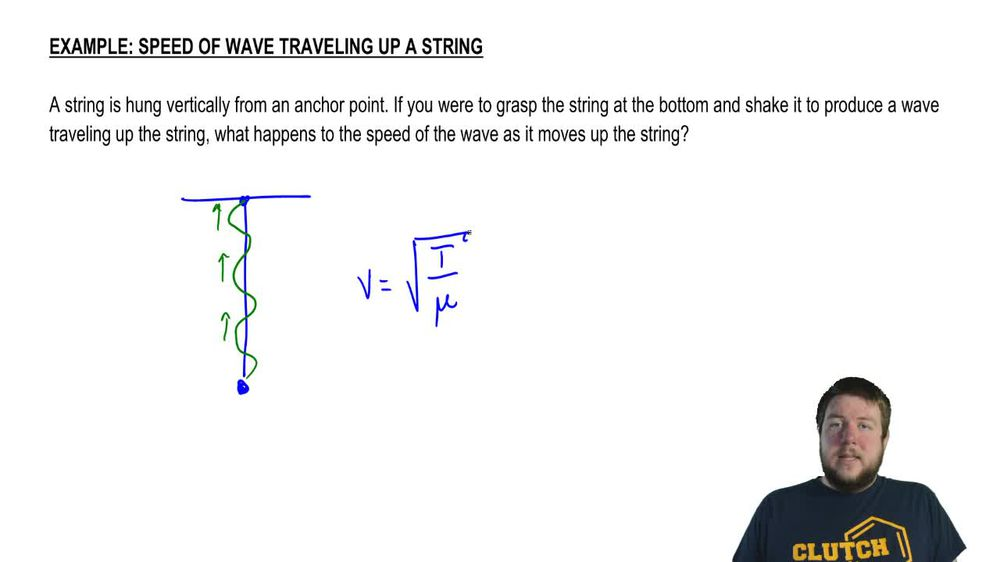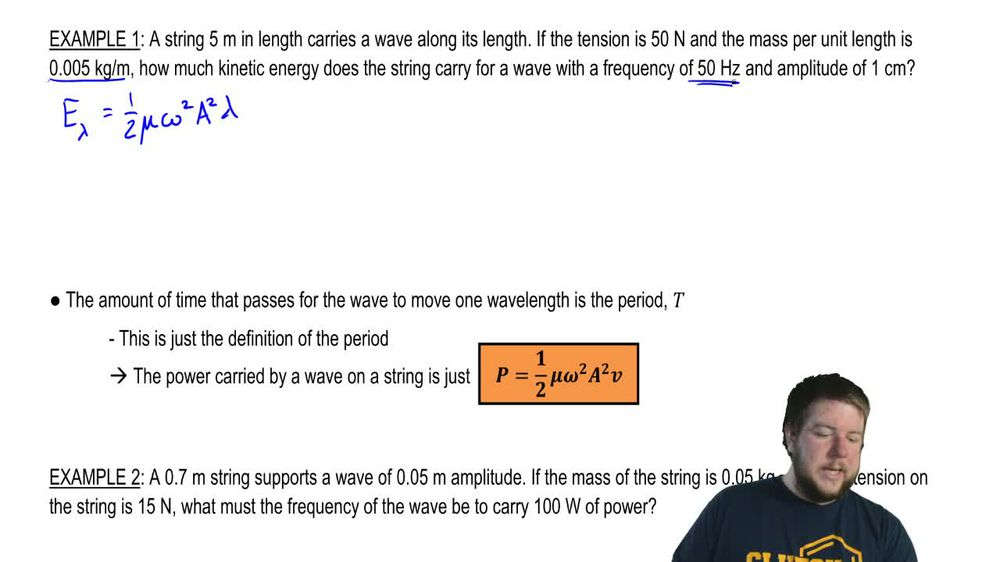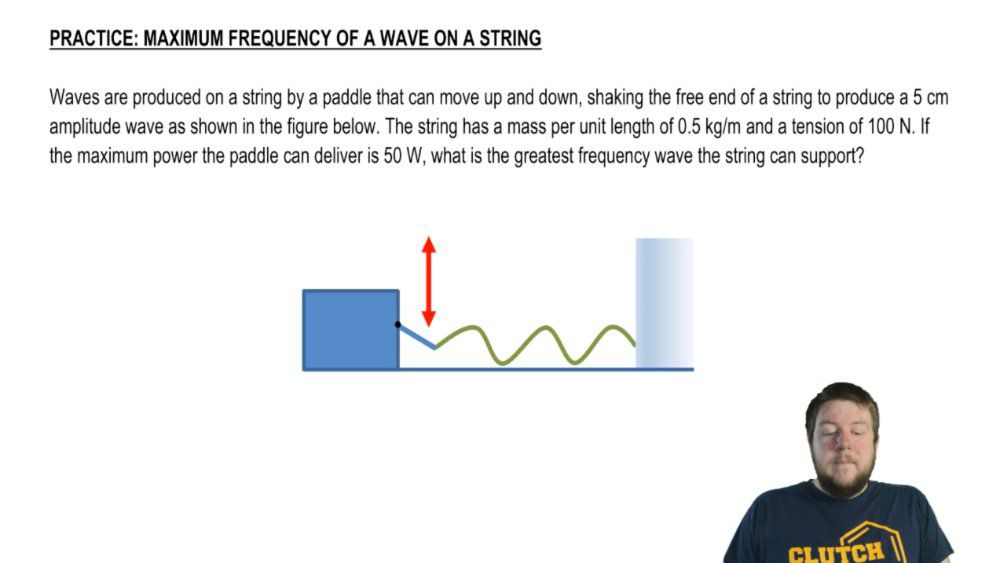Start typing, then use the up and down arrows to select an option from the list.
1. 18. Waves & Sound2. Velocity of Transverse Waves (Strings)# Maximum Frequency Of A Wave On A String

by Patrick Ford
71 views
1
0
Hey, guys, I hope you're able to solve this problem on your own. If not, here is a little bit of help. Waves air produced on a string by a paddle that can move up and down, shaking the friend of a string to produce a five centimeter amplitude wave. As shown in the figure below the string is a mass per unit length of half a kilogram per meter and attention of 100 Newtons. If the maximum power the paddle can deliver is 50 watts. What is the greatest frequency? The Sorry. What is the greatest frequency wave the string can support? Okay, so remember that power carried by a wave on a string is one half times mu omega squared a squared V. So if we want to know frequency, we'll solve for omega squared, multiply the two up to the numerator. Everything else goes to the nominator and then take the square root of both sides. Okay? We're told the maximum power we're told the mass prima length, we're told the amplitude. Okay, All we're missing is the speed of this wave. Now, this is a wave on a string, so the speed is just gonna be the square root of the tension divided by the mass period at length. We're told that the tensions 100 Newtons. So this is 100 divided by half a kilogram per meter and that speed is meters per second. Now we know everything we need to solve for that angular frequency. This is two times the power which we said was 50 watts divided by the mass screen at length, which is half a kilogram per meter. The amplitude was five centimeters or 05 m squared in the speed which was 14 m per second. And this whole thing is going to be 76 inverse seconds. But we're not done. Remember, we're looking for the greatest frequency that means linear frequencies. So we need one more step which says that the linear frequency is Omega divided by two pi which is 76 in for seconds over two pi, which is going to be 12 hurts. All right. And that wraps up this problem. Thanks for watching guys